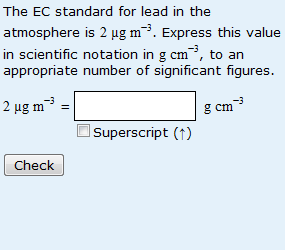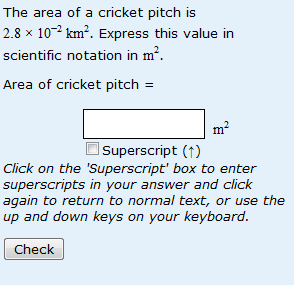# Unit conversions

As previously discussed, students aren’t great at giving correct units with their answers. However they have real probems with unit conversions!The unit conversion shown on the left  is exceptionally badly answered, and a lot of students give up without even trying. The difficulties appear to stem from different aspects of the problem, as exemplified by the examples below.

First of all, let’s look at the problem students have in converting fromone square (or cubic) unit to another, as on the right-hand side. Errors in this question fall into three basic categories:

(1) students who convert from km to m rather than from km^2 to m^2.

(2) students who do the converstion the wrong way round (i.e. convert from m^2 to km^2).

(3) students who do both of the above (i.e convert from m to km) – actually more common that just doing the conversion the wrong way round.

I’ve known for ages about the difficultly some people have in converting squared or cubic units. It fascinates me. I think it might be the result of quite a deep conceptual (mis)understanding. I think it may be linked to the fact that some people don’t really understand squaring something is not the same as multiplying it by two and that cubing it is not the same as multiplying by three.The other aspect that causes particular problems is exemplified in the conversion from ‘per hour’ to ‘per second’ in the example on the right hand side. Students can do the part of the conversion from km to m easily, but lots multiply by 3600 rather than dividing. I think this is down to problems with reciprocals (again!) and a failure to use common sense, in this case a failure to stop and think that an object or substance is likely to travel further in an hour than in a second.

This entry was posted in mathematical misunderstandings, question analysis, unit conversions and tagged , , , . Bookmark the permalink.